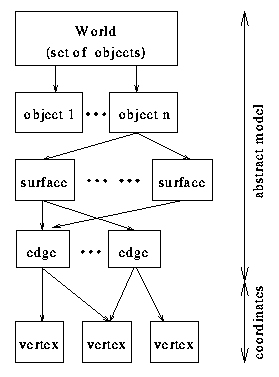Previous: Acquisition of Depth Up: 3D-Modeling for the Analysis of Range Next: Edges

# Geometric Model

The geometric model, the abstract world, is a description of a set of objects.Figure 4: Motivating the model by detectable primitives

The structure of a model depends on its intention. In our case it is an abstract description of those parts of the real world objects, which can easily be detected in the range data. The grey-filled boxes in Figure 4 represent the knowledge we got by analyzing the range image. We are able to detect this primitives. Now object recognition is the determination to which object the found structures belong.Figure 5: The basic structure of the modeling conception

The modeled world is a set of objects, which are made of surfaces, edges, vertices and neighborhood relations between surfaces. The basic structure of the model is a tree (Figure 5). Figure 5 is very similar to Figure 4 because

• it is necessary to model anything we can detect,
• it is not useful to model anything else, because of runtime memory, time for modeling and so forth.
The nodes of the tree are geometric elements like edges and surfaces. The edges of the tree represent the relation "belongs to". Vertices belong to edges, edges belong to surfaces and surfaces belong to objects. When an edge belongs to two different surfaces, this fact implicates neighborhood of those surfaces. The tree itself is thus a representation of the structure of an object. The leaves are vertices and consist of three dimensional coordinates. Each object has its own object coordinate system (OCS) and all vertices are defined in this coordinate system (Figure 8).Previous: Acquisition of Depth Up: 3D-Modeling for the Analysis of Range Next: Edges

Last updated 1997/07/11, © Jochen Duckeck